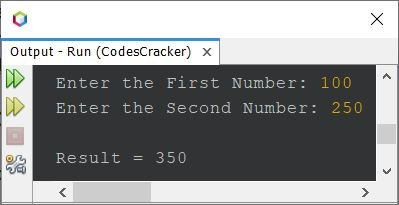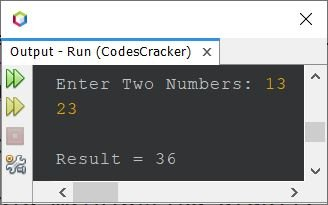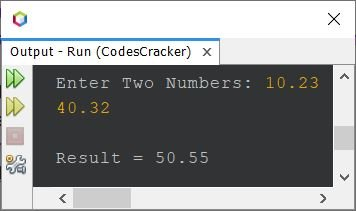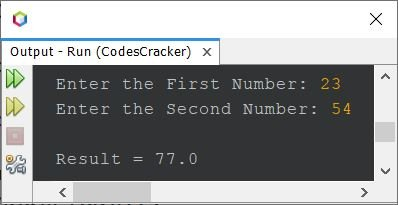# Java Program to Add Two Numbers

• Simplest program of adding two numbers in Java
• Add two numbers using Scanner (or by user-input)
• Add two numbers using user-defined function
• Add two numbers using class
• Add two numbers using constructor

## Add Two Numbers in Java - Simplest Version

The question is, write a Java program to add two numbers. The program given below is the answer to this question:

```import java.util.Scanner;

public class CodesCracker
{
public static void main(String[] args)
{
int numberOne = 5, numberTwo = 10, add;

}
}```

The output produced by above Java program is:

`Result = 15`

Now let's move on, and create a program in Java that takes inputs from user and then adds that given two numbers.

## Add Two Numbers in Java using Scanner

The question is, write a Java program to add any two numbers. Both the number must be received by user at run-time of the program. The program given below is its answer:

```import java.util.Scanner;

public class CodesCracker
{
public static void main(String[] args)
{
Scanner s = new Scanner(System.in);

System.out.print("Enter the First Number: ");
numberOne = s.nextInt();
System.out.print("Enter the Second Number: ");
numberTwo = s.nextInt();

}
}```

The snapshot given below shows the sample run of above program, with user input 100 and 250 as two numbers:The above program can also be created in this way:

```import java.util.Scanner;

public class CodesCracker
{
public static void main(String[] args)
{
Scanner s = new Scanner(System.in);

System.out.print("Enter Two Numbers: ");
int a = s.nextInt();
int b = s.nextInt();

System.out.println("\nResult = " +(a+b));
}
}```

The snapshot given below shows the sample run of above Java program with user input 13 and 23 as two numbers:There is a limitation with above program. That limitation is, the program only works with integer. Therefore let me create another program that works for both integer and real numbers:

```import java.util.Scanner;

public class CodesCracker
{
public static void main(String[] args)
{
Scanner s = new Scanner(System.in);

System.out.print("Enter Two Numbers: ");
float a = s.nextFloat();
float b = s.nextFloat();

System.out.println("\nResult = " +(a+b));
}
}```

Here is its sample run with user input 10.23 and 40.32 as two numbers:## Add Two Numbers in Java using Function

This program is created to show, how the addition of two numbers can be performed in Java using a user-defined function. That is, a function named add() is created, that takes two arguments and returns the addition result of its arguments.

```import java.util.Scanner;

public class CodesCracker
{
public static void main(String[] args)
{
Scanner s = new Scanner(System.in);

System.out.print("Enter the First Number: ");
float a = s.nextFloat();
System.out.print("Enter the Second Number: ");
float b = s.nextFloat();

}

public static float add(float x, float y)
{
return (x+y);
}
}```

The sample run of above program is:## Add Two Numbers in Java using Class

Here is another program created using a class. That is, an object obj of the class CodesCracker is created. And using the object obj, we can call the method add() of the class. Rest of the things are similar to previous program, that was created using function.

```import java.util.Scanner;

public class CodesCracker
{
static int add(int x, int y)
{
return (x+y);
}
public static void main(String[] args)
{
Scanner s = new Scanner(System.in);

System.out.print("Enter the First Number: ");
int a = s.nextInt();
System.out.print("Enter the Second Number: ");
int b = s.nextInt();

CodesCracker obj = new CodesCracker();

System.out.println("\nResult = " +res);
}
}```

## Add Two Numbers in Java using Constructor

This is the last program of this article, created using a constructor. A constructor is basically a method having its name, same as the name of the class. Constructor gets automatically executed when an object of the class will get created.

```import java.util.Scanner;

public class CodesCracker
{
CodesCracker(int x, int y)
{
}
public static void main(String[] args)
{
Scanner s = new Scanner(System.in);

System.out.print("Enter the First Number: ");
int a = s.nextInt();
System.out.print("Enter the Second Number: ");
int b = s.nextInt();

CodesCracker obj = new CodesCracker(a, b);
}
}```

The above program produces similar result/output as of previous program. In above program, after executing the statement:

`CodesCracker obj = new CodesCracker(a, b);`

The constructor (a method inside the class with its name, same as class name) named CodesCracker() automatically gets executed. That is the value of a and b gets passed to x and y, the two parameters of the constructor. And the addition result of these two numbers gets initialized to add variable. Finally the value of add gets printed on output using the statement:

`System.out.println("\nResult = " +add);`

available inside the constructor as its second statement.

#### Same Program in Other Languages

Java Online Test

« Previous Program Next Program »